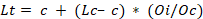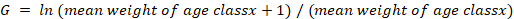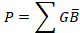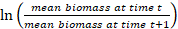# Data: Diversity-production relationships of fish communities in freshwater stream ecosystems

## Cite this dataset

Myers, Bonnie (2021). Data: Diversity-production relationships of fish communities in freshwater stream ecosystems [Dataset]. Dryad. https://doi.org/10.5061/dryad.0rxwdbs0s

## Abstract

Ecological relationships between species richness and biomass production are increasingly thought to be pervasive across the globe. Yet diversity-production relationships have not been explored extensively for freshwater fish communities even though fisheries production provides key services to humans. Our aim was to evaluate the diversity-production relationship for fish communities inhabiting freshwater streams across the Appalachian Mountain range and examine how diversity-production relationships varied across streams possessing different thermal signatures. Our study area included 25 freshwater stream ecosystems spanning from Vermont to North Carolina in the United States. Twenty sites were located in Maryland south to Tennessee and North Carolina while five additional higher latitude sites were sampled in Massachusetts and Maine. We sampled 25 study streams from June to September 2012 and collected fish population information to calculate biomass, species richness, Shannon diversity index, and annual production for each fish community. Linear mixed models were used to analyze the relationship between diversity indices and total community production. We also compared diversity and production relationships across other taxa. Across all streams, community fish production, biomass and P/B ratios ranged 0.15-6.79 g m2 y1, 0.61-0.73 g m-2, and 0.21-1.07 y-1, respectively. Species richness had a significant positive effect (p = 0.012) on community fish production, while accounting for the thermal signature of the streams as a random effect and other habitat covariates. Shannon diversity index did not have a significant effect (p = 0.101) on community production. The diversity-production relationship observed for stream fish communities was similar to other studies but demonstrated one of the highest slopes. Our results demonstrate that effects of biodiversity resonate to influence the production of fishes; thus, management of fisheries is more closely coupled to biodiversity than previously thought.

## Methods

Data Collection.—All data collection occurred from June-September 2012 except for field collection of air and water temperature data, which was continuously downloaded biannually following deployment. A random starting point upstream of the air and water temperature loggers was selected for each stream site. Using a systematic sampling design, ETS Electrofishing, LLC ABP-3Q-600 volt backpack electrofishing units were used to sample fish from two 50 meter stream reaches spaced 50 meters apart until depletion of the sampling area (e.g., 3-5 passes per reach) Block nets with 1/16’’ mesh were placed downstream and upstream of each 50 meter reach to inhibit immigration and emigration of fishes within the sampling area. For all individuals captured, total lengths (mm) and weights (g) were measured. Sub-samples of each species captured at each site were euthanized in tricaine methanesulfonate (MS-222) and frozen for examination and age estimation in the lab. Following Ketchen (1950) and Devries and Frie (1996) sub-sampling protocol, a fixed stratified sub-sample of approximately 10 individuals per species per length group was collected. To reduce sampling bias, length-group intervals were relatively small (i.e., 30-40 mm, 40-50 mm, 50-60 mm) (Devries & Frie, 1996).

Data Processing.—Species richness was determined for each site as the total number of unique species, and mean species richness was calculated for the four site temperature classes (i.e., cold-, cool-, warm-, and northern cold-water). In addition, α-diversity was calculated using the Shannon diversity index (Colwell, 2009). We used species richness and Shannon diversity index to investigate the production relationship with both the raw number of species present (i.e., species richness) and the evenness among species (i.e., Shannon diversity index) (Colwell, 2009).

Sagittal otoliths were extracted from each retained specimen for estimation of age-at-capture and back-calculation of lengths at previous ages (Devries & Frie, 1996). Putative annual growth rings were counted under a microscope and interannual growth increments measured by an experienced reader using a computer-based image analysis system interfaced with the microscope. Length-at-previous ages of all fish were calculated using the Fraser-Lee equation (Francis, 1990)

Lt= c + (Lc– c) * (Oi/Oc)where Lt=back calculated length at ith annuli, c=intercept constant, Lc=length of fish at capture, Oi=otolith radius at ith annuli, and Oc=otolith radius at capture (Francis, 1990; DeVries & Fries, 1996). The intercept for each species was determined by plotting fish length as a function of otolith radius length (Francis, 1990). Finally, logarithmic or power growth functions (depending on the strength of the function fit) were used to predict the ages of measured fish of unknown age using total length data as a predictor.

Secondary production values for each species were estimated using the instantaneous growth rate method (Waters, 1977; Hayes, Bence, Kwak, & Thompson, 2007). Age-specific growth (G) was calculated using the equation below.

G = ln (mean weight of age classx+1) / (mean weight of age classx)Age-specific biomass was calculated as the sum of the weights of all individuals sampled within that age class. Age-specific production was then estimated as the production of age-specific biomass and growth (Halyk & Balon, 1983; Valentine-Rose, Layman, Arrington, & Rypel, 2007; Valentine-Rose, Rypel, & Layman, 2011; Rypel, Goto, Sass, & Vander Zanden, 2015). This method integrates the area under the traditional Allen curve with fish age classes on the x-axis and mean weight on the y-axis (Allen, 1949; Hayes et al., 2007). The formal equation used to calculate production was

P=GBwhere P=production, B=arithmetic mean standing stock, and G=instantaneous growth rate (i.e, lnmean biomass at time tmean biomass at time t+1(Rypel et al., 2015). Annual production (g m-2 y-1) for each species was calculated as the sum of production between each age class (Halyk & Balon, 1983), and whole community fish production was calculated by summing all individual species annual production values for the community (Halyk & Balon, 1983). The P/B ratio for each species was calculated as annual production divided by mean annual biomass as an estimate of the estimated biomass turnover rate for that species in each stream (Waters, 1977; Hayes et al., 2007). Community P/B was calculated by dividing total community production by the total community biomass.

Species richness was determined for each site as the total number of unique species, and mean species richness was calculated for the four site temperature classes (i.e., cold-, cool-, warm-, and northern cold-water). In addition, α-diversity was calculated using the Shannon diversity index (Colwell, 2009).We used species richness and Shannon diversity index to investigate the production relationship with both the raw number of species present (i.e., species richness) and the evenness among species (i.e., Shannon diversity index) (Colwell, 2009).

Sagittal otoliths were extracted from each retained specimen for estimation of age-at-capture and back-calculation of lengths at previous ages (Devries & Frie, 1996). Putative annual growth rings were counted under a microscope and interannual growth increments measured by an experienced reader using a computer-based image analysis system interfaced with the microscope. Length-at-previous ages of all fish were calculated using the Fraser-Lee equation (Francis, 1990)

Lt= c + (Lc– c) * (Oi/Oc)where Lt=back calculated length at ith annuli, c=intercept constant, Lc=length of fish at capture, Oi=otolith radius at ith annuli, and Oc=otolith radius at capture (Francis, 1990; DeVries & Fries, 1996). The intercept for each species was determined by plotting fish length as a function of otolith radius length (Francis, 1990). Finally, logarithmic or power growth functions (depending on the strength of the function fit) were used to predict the ages of measured fish of unknown age using total length data as a predictor.

Secondary production values for each species were estimated using the instantaneous growth rate method (Waters, 1977; Hayes, Bence, Kwak, & Thompson, 2007). Age-specific growth (G) was calculated using the equation below.

G = ln (mean weight of age classx+1) / (mean weight of age classx)Age-specific biomass was calculated as the sum of the weights of all individuals sampled within that age class. Age-specific production was then estimated as the production of age-specific biomass and growth (Halyk & Balon, 1983; Valentine-Rose, Layman, Arrington, & Rypel, 2007; Valentine-Rose, Rypel, & Layman, 2011; Rypel, Goto, Sass, & Vander Zanden, 2015). This method integrates the area under the traditional Allen curve with fish age classes on the x-axis and mean weight on the y-axis (Allen, 1949; Hayes et al., 2007). The formal equation used to calculate production was

P=GBwhere P=production, B=arithmetic mean standing stock, and G=instantaneous growth rate (i.e, lnmean biomass at time tmean biomass at time t+1(Rypel et al., 2015). Annual production (g m-2 y-1) for each species was calculated as the sum of production between each age class (Halyk & Balon, 1983), and whole community fish production was calculated by summing all individual species annual production values for the community (Halyk & Balon, 1983). The P/B ratio for each species was calculated as annual production divided by mean annual biomass as an estimate of the estimated biomass turnover rate for that species in each stream (Waters, 1977; Hayes et al., 2007). Community P/B was calculated by dividing total community production by the total community biomass.

## Usage notes

This dataset contains the final fish community values (e.g., production, species diversity indices, etc.) and associated habitat covariates that were used in the manuscript.

## Funding

College of Agriculture and Life Sciences, Virginia Polytechnic Institute and State University

U.S. Forest Service